# Week 1 - lecture 2

## TOP TOPIC

Date: 01/09/22

1.6. Reversibility and Time Reversals
1.7. Eulerian graphs
1.8. Harmonic functions
1.9. Gambler's Ruin

## Homework

1.  A  connected graph is a tree if it contains no cycles. Show that every finite tree with at least two vertices must have at least two leaves. (a leaf is a vertex of degree 1).
2.  A vertex coloring of a graph is called proper if every pair of  adjacent vertices are assigned different colors. Consider the following Markov chain on the set of proper 3 colorings of a finite tree: At each step, a vertex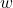ofis selected uniformly at random, and is assigned randomly, uniformly  one of the colors that does not appear among the neighbors of. Show that this chain is irreducible.
3.  Letbe a transition matrix on a finite set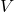. Suppose that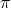is a stationary distribution forand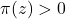. Show  that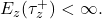## Reference

 Glasner, Shmuel.
Almost periodic sets and measures on the torus.
Israel J. Math. 32 (1979), no. 2-3, 161–-172.

 Berend, Daniel and Peres, Yuval.
Asymptotically dense dilations of sets on the circle.
J. London Math. Soc. (2) 47 (1993), no. 1, 1–-17.

 N. Alon and Y. Peres, "Uniform dilations'', Geometric and Functional Analysis, vol. 2, No. 1 (1992), 1–28.

 Nair, R. and Velani, S. L.
Glasner sets and polynomials in primes.
Proc. Amer. Math. Soc. 126 (1998), no. 10, 2835–-2840.